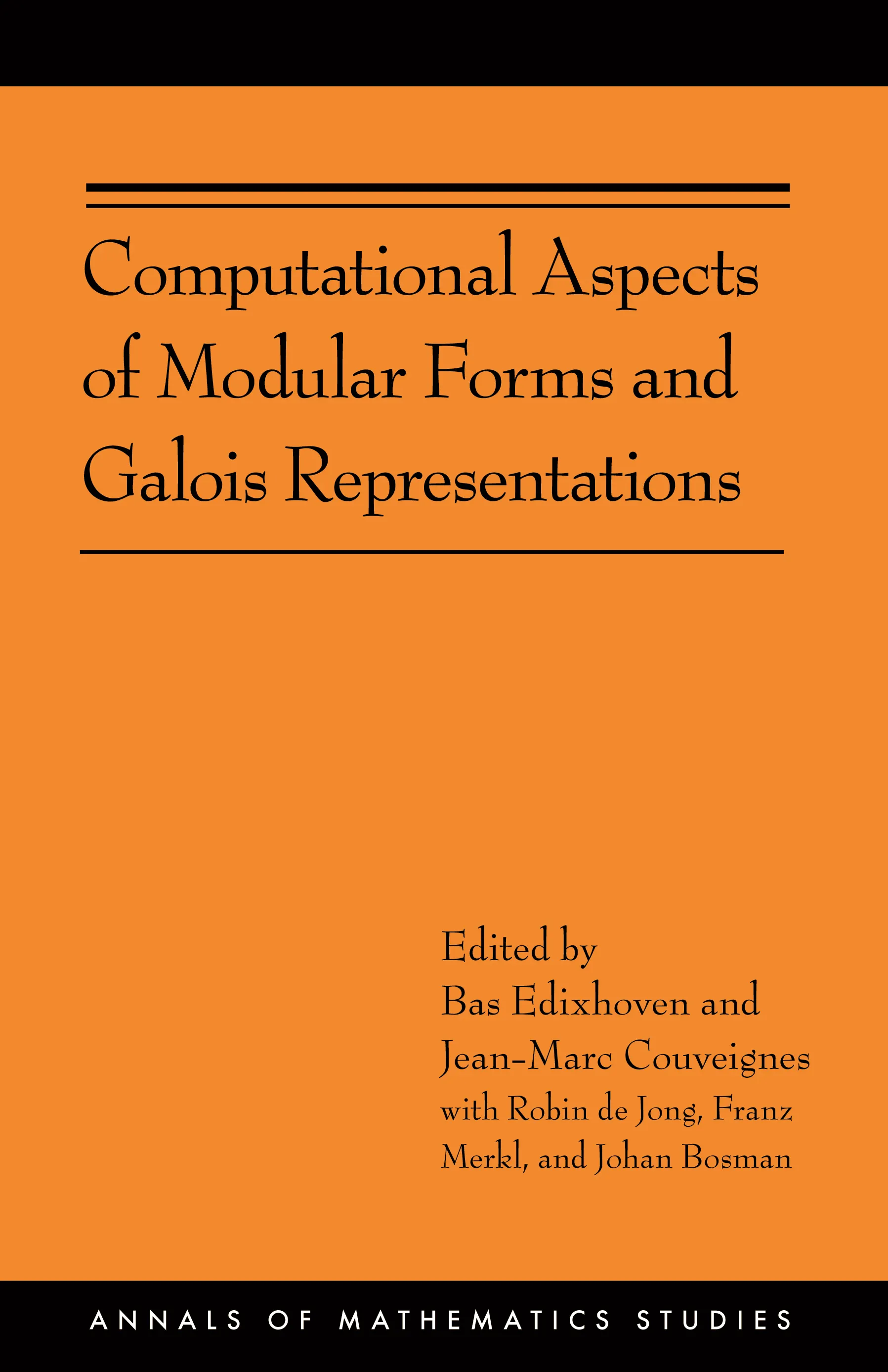#### Computational Aspects of Modular Forms and Galois Representations

Bas Edixhoven

##### Before you purchase audiobooks and ebooks

Please note that audiobooks and ebooks purchased from this site must be accessed on the Princeton University Press app. After you make your purchase, you will receive an email with instructions on how to download the app.

# Computational Aspects of Modular Forms and Galois Representations: How One Can Compute in Polynomial Time the Value of Ramanujan's Tau at a Prime (AM-176)

Contributions by
• Robin de Jong
• Franz Merkl
• Johan Bosman## Paperback

Price:
\$95.00/£80.00
ISBN:
Published:
Jun 20, 2011
2011
Pages:
440
Size:
6 x 9.25 in.
Illus:
6 line illus.

Modular forms are tremendously important in various areas of mathematics, from number theory and algebraic geometry to combinatorics and lattices. Their Fourier coefficients, with Ramanujan’s tau-function as a typical example, have deep arithmetic significance. Prior to this book, the fastest known algorithms for computing these Fourier coefficients took exponential time, except in some special cases. The case of elliptic curves (Schoof’s algorithm) was at the birth of elliptic curve cryptography around 1985. This book gives an algorithm for computing coefficients of modular forms of level one in polynomial time. For example, Ramanujan’s tau of a prime number p can be computed in time bounded by a fixed power of the logarithm of p. Such fast computation of Fourier coefficients is itself based on the main result of the book: the computation, in polynomial time, of Galois representations over finite fields attached to modular forms by the Langlands program. Because these Galois representations typically have a nonsolvable image, this result is a major step forward from explicit class field theory, and it could be described as the start of the explicit Langlands program.

The computation of the Galois representations uses their realization, following Shimura and Deligne, in the torsion subgroup of Jacobian varieties of modular curves. The main challenge is then to perform the necessary computations in time polynomial in the dimension of these highly nonlinear algebraic varieties. Exact computations involving systems of polynomial equations in many variables take exponential time. This is avoided by numerical approximations with a precision that suffices to derive exact results from them. Bounds for the required precision — in other words, bounds for the height of the rational numbers that describe the Galois representation to be computed — are obtained from Arakelov theory. Two types of approximations are treated: one using complex uniformization and another one using geometry over finite fields.

The book begins with a concise and concrete introduction that makes its accessible to readers without an extensive background in arithmetic geometry. And the book includes a chapter that describes actual computations.